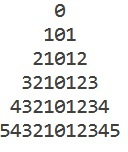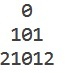# C++ Program for triangular pattern (mirror image around 0)

Given with the positive value n and the task is to generate the triangular pattern i.e. mirror image of the printed numbers and display the result

## Example

Input-: n = 6
Output-:Input-: n = 3
Output-:Approach used in the below program is as follows

• Input the value of n as a positive integer
• Traverse one loop i for the number of rows in a pattern i.e. n
• Traverse one loop j for the number of spaces in a pattern
• Traverse another loop for the digits in a pattern

## Algorithm

START
Step 1-> declare function to print mirror image of triangular pattern
void print_mirror(int n)
declare and set int temp = 1 and temp2 = 1
Loop for int i = 0 and i < n and i++
Loop For int j = n - 1 and j > i and j—
print space
End
Loop For int k = 1 and k <= temp and k++
print abs(k - temp2)
End
Set temp += 2
increment temp2++
print \n
Step 2-> In main()
Declare int n = 6
print_mirror(n)
STOP

## Example

#include <bits/stdc++.h>
using namespace std;
//function to print mirror image of triangular pattern
void print_mirror(int n) {
int temp = 1, temp2 = 1;
for (int i = 0; i < n; i++) {
for (int j = n - 1; j > i; j--) {
cout << " ";
}
for (int k = 1; k <= temp; k++) {
cout << abs(k - temp2);
}
temp += 2;
temp2++;
cout << "\n";
}
}
int main() {
int n = 6;
print_mirror(n);
return 0;
}

## Output